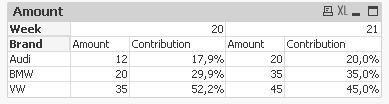QlikView App Development

Discussion Board for collaboration related to QlikView App Development.

Announcements
QlikWorld 2020: Join us May 11 - 14, 2020 in Phoenix, AZ. Register early and save \$400. Learn MoreNot applicable

Divided by dimension total

Hi,

I am trying to figure something out.

i have a sales case in which i sell cars. i have VW, BMW and Audi's.

every week i get a report on the sales from this week. i can see which cars are selling the best each week.

i can also see the contribution of each car in %

for example

week 20

BrandAmountContribution
Audi1218%
BMW2030%
VW3552%
Total67100%

The above table is easy to replicate in QV.

But now i want to see all weeks in the same table but i want to see the contribution of a Brand for each week and not the contribution on the year total.

WeekBrandAmountContribution
20Audi1218%
20BMW2030%
20VW3552%
21Audi2020%
21BMW3535%
21VW4545%

is it possible to create it like that?

1 Solution

Accepted SolutionsPartner

Re: Divided by dimension total

try in the table and show in percentage:

contribution: sum(Amount)/sum(total <Week> Amount)

9 RepliesLuminary

Re: Divided by dimension total

You need to create Week-field, ideally by using the following expression: week('Date') as Week.Not applicable

Re: Divided by dimension total

I have a master calendar which contains a week-field. that is not my question.

I want to know if it is possible to replicate my table above.

The % is calculated in the table and not a value in my datasourcePartner

Re: Divided by dimension total

try in the table and show in percentage:

contribution: sum(Amount)/sum(total <Week> Amount)MVP

Re: Divided by dimension total

Nard

You'll need to use the total qualifier in your expression.  The Chart Aggregation functions section of the Help describes it at length.Partner

Re: Divided by dimension total

Hi:

This is possible using your week as a calculated dimension.Partner

Re: Divided by dimension total

How about something like this:

avg(aggr(nodistinct sum(Amount)/sum(Total <Week> Amount),Week,Brand))Partner

Re: Divided by dimension total

Hi,

you can create a straight table with week (or weekyear) and brand as dimensions. Also you can have the same amount expression as in your example. What will change is that your expression for the weekly contribution per brand has to be something like this:

sum(Amount) / sum(total <week> Amount)

of course, you may add any additional filter (with set analysis or direct selections) so you can get to the result you need,

hope this helps,

regardsMVP

Re: Divided by dimension total

Hi,

one example:hope this helps

regards

MarcoNot applicable

Re: Divided by dimension total

Yes this works perfect, thank you